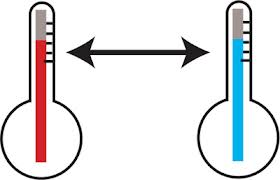# The Heat is On

Algebra Level 2Satvik and Agnishom are scientists collaborating on a thermodynamic experiment. Both use their own scales to measure temperature.

On Satvik's scale, freezing point of water is $-30$ degrees $S$ and the boiling point of water is $90$ degrees $S$.

On Agnishom's scale the corresponding readings are $20$ and $100$ degrees $A$ respectively.

At what temperature will both these scales show the same reading in $S$ and $A$ degrees?

×